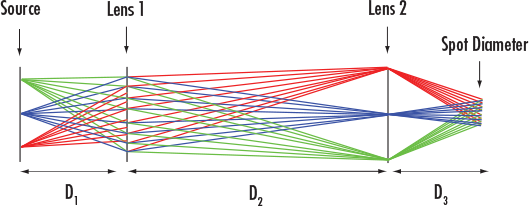# Koehler Illumination Calculator

View All Technical Tools

Source Diameter (mm):
Lens 1 Diameter (mm):
Lens 1 Focal Length (mm):
Lens 2 Diameter (mm):
Lens 2 Focal Length (mm):

Source to Lens 1 Distance (mm): --

Lens 1 to Lens 2 Distance (mm): --

Lens 2 to Spot Diameter Distance (mm): --

System Length (mm): --

Spot Diameter (mm): --

Input Numerical Aperture, NA: --

Output Numerical Aperture, NA: --$$d_1 = \text{FL}_1 \left[ \frac{D_{\text{Source}} + D_{L_2}}{D_{L_2}} \right]$$
$$d_2 = \frac{d_1 \, \text{FL}_1}{d_1 - \text{FL}_1}$$
$$d_3 = \frac{d_2 \, \text{FL}_2}{d_2 - \text{FL}_2}$$
$$\text{System Length} = d_1 + d_2 + d_3$$
$$D_{\text{Spot}} = \frac{D_{L_1} \, \text{FL}_2}{d_2 - \text{FL}_2}$$
$$\text{NA}_{\text{Input}} = \sin{\left[ \tan^{-1}{\left( \frac{D_{L_1}}{2 d_1} \right)} \right]}$$
$$\text{NA}_{\text{Output}} = \sin{\left[ \tan^{-1}{\left( \frac{D_{L_2}}{2 d_3} \right)} \right]}$$
 d1 Source to Lens 1 Distance d2 Lens 1 to Lens 2 Distance d3 Lens 2 to Spot Distance DL1 Diameter of Lens 1 DL2 Diameter of Lens 2 FL1 Focal Length of Lens 1
 FL2 Focal Length of Lens 2 Dsource Source Diameter Dspot Spot Diameter NAinput Input Numerical Aperture NAoutput Output Numerical Aperture

## Description

Mathematically determine how to configure a source and two lenses into a Koehler Illumination setup. Calculator uses the paraxial lens approximation and enables an initial lens selection. Once lenses are selected, EO recommends modeling one's Koehler Illumination setup in optical design software such as Zemax, CodeV, or FRED to analyze and revise as needed. Please note that performance will vary based on source and lens selections.

View All Now